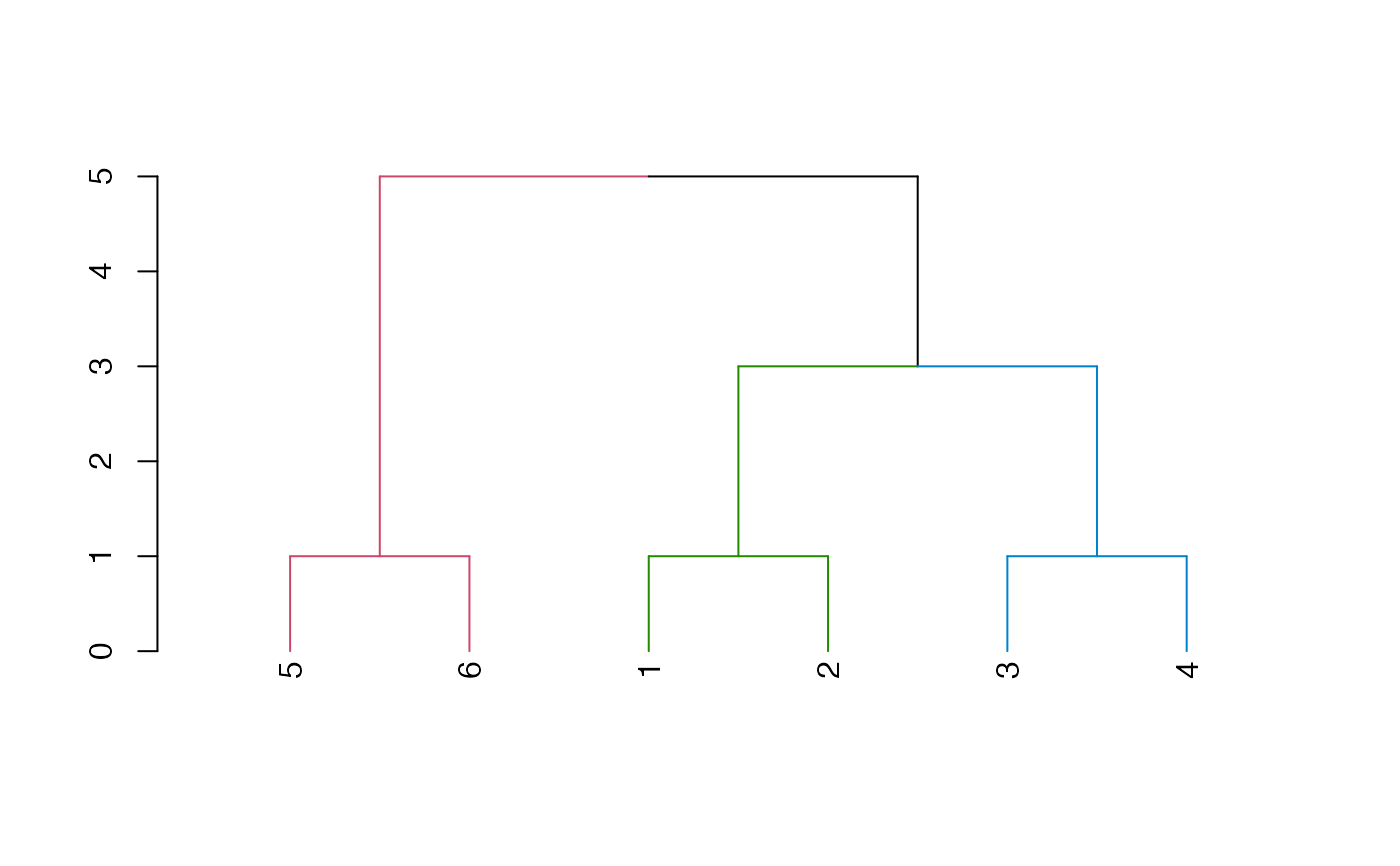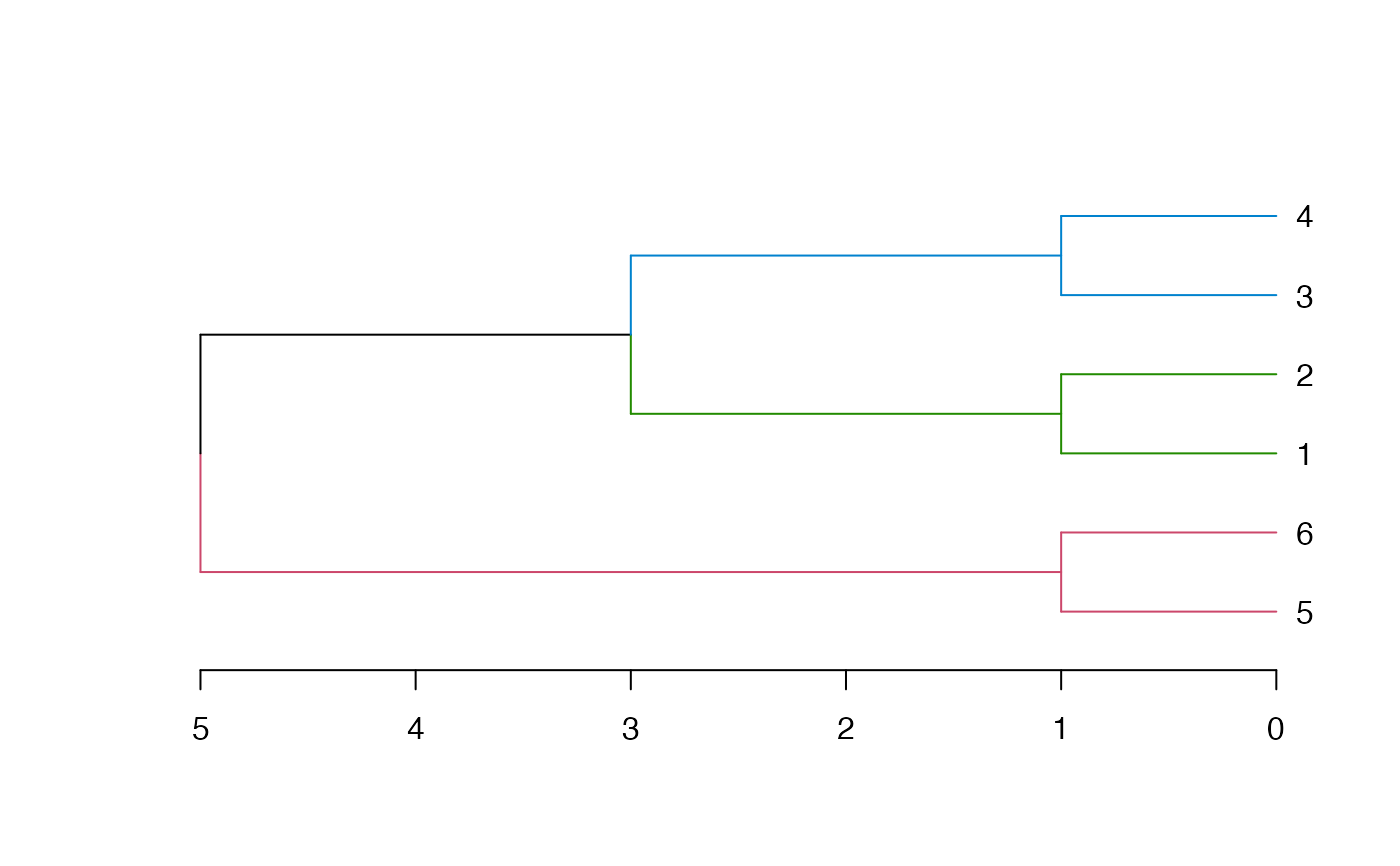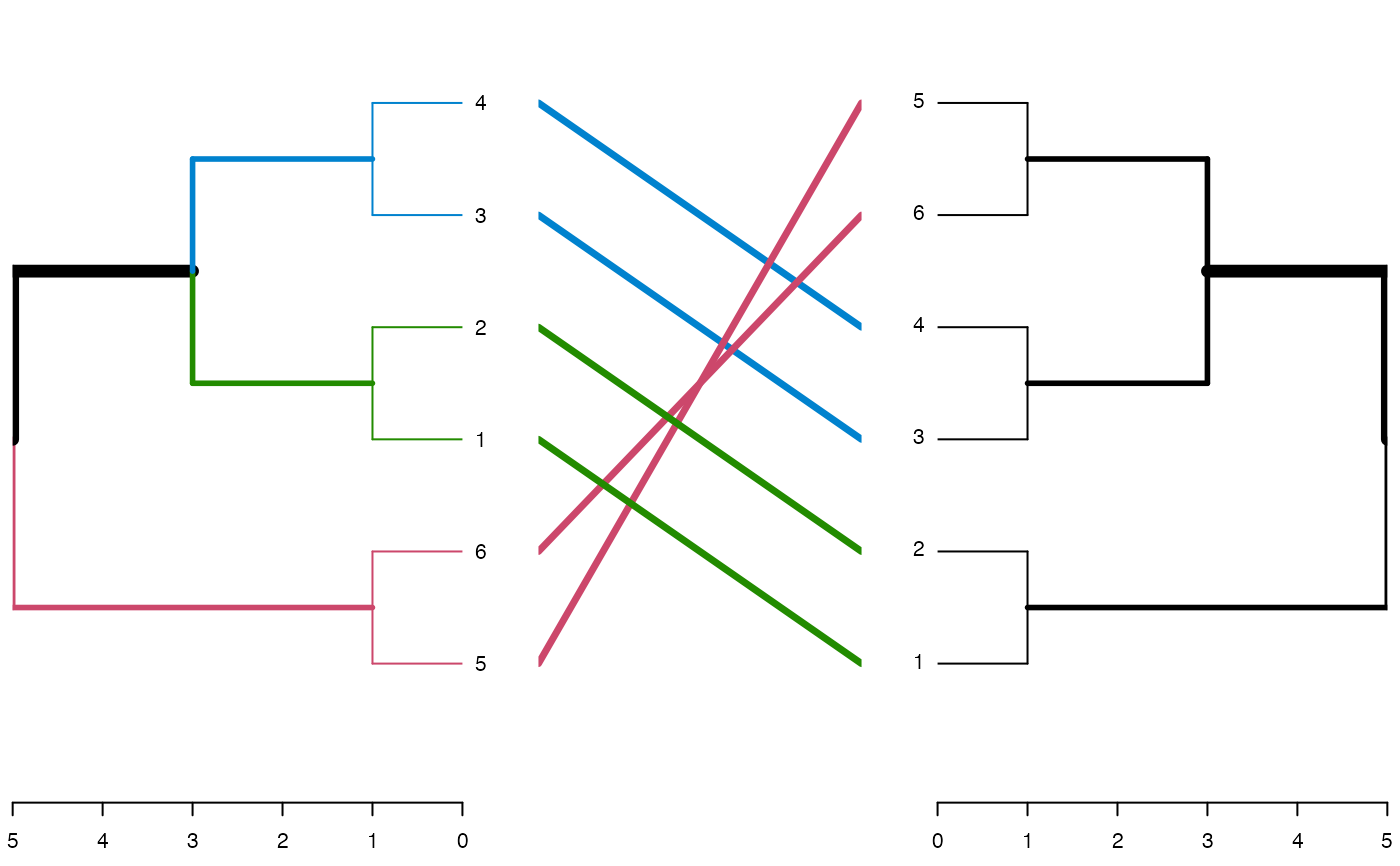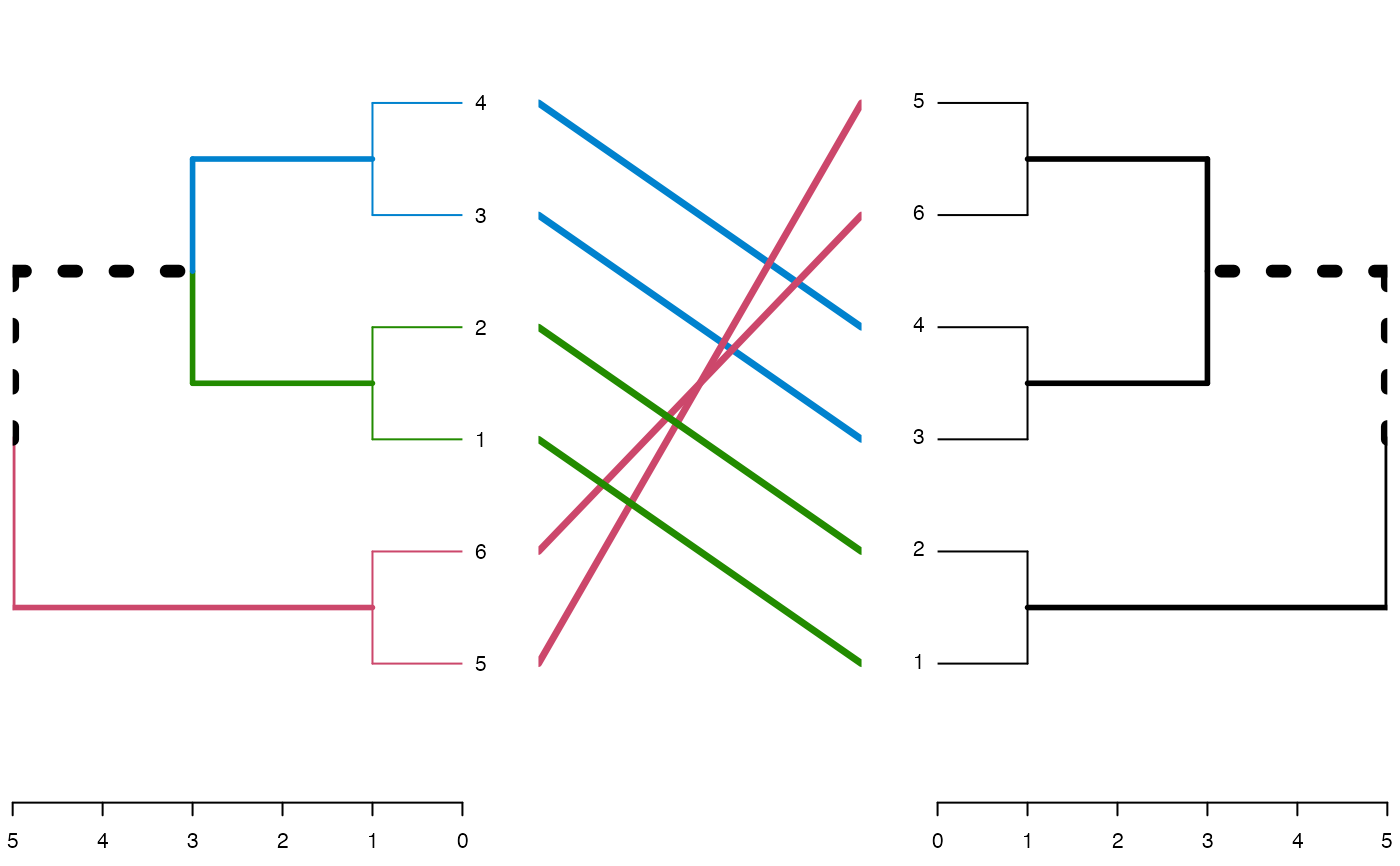Gets a dend and the output from "nodes_with_shared_labels" and returns a vector (length of labels), indicating the clusters forming shared subtrees

common_subtrees_clusters(dend1, dend2, leaves_get_0_cluster = TRUE, ...)

## Arguments

dend1
dend2
leaves_get_0_cluster

logical (TRUE). Should the leaves which are not part of a larger common subtree get a unique cluster number, or the value 0.

...

not used.

## Value

An integer vector, with values indicating which leaves in dend1 form a common subtree cluster, with ones available in dend2

## Examples



library(dendextend)
dend1 <- 1:6 %>%
dist() %>%
hclust() %>%
as.dendrogram()
dend2 <- dend1 %>% set("labels", c(1:4, 6:5))
tanglegram(dend1, dend2)clusters1 <- common_subtrees_clusters(dend1, dend2)
dend1_2 <- color_branches(dend1, clusters = clusters1)
plot(dend1_2)plot(dend1_2, horiz = TRUE)tanglegram(dend1_2, dend2, highlight_distinct_edges = FALSE)tanglegram(dend1_2, dend2)